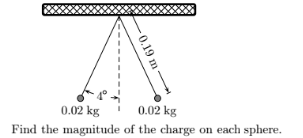Physics Practice Problems Coulomb's Law (Electric Force) Practice Problems Solution: Two identical small charged spheres hang in equili...

🤓 Based on our data, we think this question is relevant for Professor Chen's class at UTA.

# Solution: Two identical small charged spheres hang in equilibrium with equal masses as shown in the figure. The length of the strings are equal and the angle (shown in the figure) with the vertical is identical.The acceleration of gravity is 9.8 m/s 2 and the value of Coulomb’s constant is 8.98755×10 9 N m2/C2.

###### Problem

Two identical small charged spheres hang in equilibrium with equal masses as shown in the figure. The length of the strings are equal and the angle (shown in the figure) with the vertical is identical.

The acceleration of gravity is 9.8 m/s and the value of Coulomb’s constant is 8.98755×10 9 N m2/C2.Coulomb's Law (Electric Force)

Coulomb's Law (Electric Force)

#### Q. Four charges are arranged as shown in the figure below each with a positive (red) charge or a negative (blue) charge. Find the net total force (magnit...

Solved • Tue Dec 27 2016 15:05:06 GMT-0500 (EST)

Coulomb's Law (Electric Force)

#### Q. A positive test charge q is released near a positive fixed charge Q. As q moves away from Q, it will move with A) increasing acceleration. B) consta...

Solved • Tue Dec 27 2016 15:01:25 GMT-0500 (EST)

Coulomb's Law (Electric Force)

#### Q. A point charge Q = -12 μC, and two other charges, q 1 and q2, are placed as shown. The electric force components on charge Q are Fx = +0.005 N and Fy ...

Solved • Mon Dec 26 2016 14:27:40 GMT-0500 (EST)

Coulomb's Law (Electric Force)

#### Q. Electrical and gravitational forces follow similar equations with one main difference: A) Gravitational forces obey the inverse square law and electr...

Solved • Mon Dec 26 2016 13:17:19 GMT-0500 (EST)